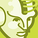# App Development

Announcements
cancel
Showing results for
Did you mean:Contributor II

## Making Filter Panes Static (?)

I'm somewhat new to Qlik Sense, having gone through training, and now building my second application.   I have two filter panes designed to show all the Sundays in current fiscal year, and previous fiscal year, respectively.   The field is "ActivityDate".  They are both working as far as showing the correct dates.  However, I want them both to ignore any other filters/selections.  I also have another filter pane on an "ActivityType" field.  I want that to ignore any other filters/selections as well.   The expressions in the filter pane dimensions are as follows:

Current Fiscal Year Sundays

= Date(Aggr(Only( { <ActivityDate={">=\$(=Timestamp(YearStart(Now(), 0,11)))<=\$(=Timestamp(Now()))"}> * <ActivityDate= {"=num(weekday(ActivityDate))=0"} >} ActivityDate), ActivityDate),'MM/DD/YYYY')

Previous Fiscal Year Sundays

Type

=aggr( only ( {<ActivityType={'Agreement TBI','Settlement'}>} if(ActivityType='Agreement TBI','Sales',ActivityType) ), ActivityType)

These expressions work fine, but when I put a 1 in front of the set, they don't ignore selections like I want them to. Examples:

= Date(Aggr(Only( { 1<ActivityDate={">=\$(=Timestamp(YearStart(Now(), 0,11)))<=\$(=Timestamp(Now()))"}> * <ActivityDate= {"=num(weekday(ActivityDate))=0"} >} ActivityDate), ActivityDate),'MM/DD/YYYY')

=aggr( only ( {1<ActivityType={'Agreement TBI','Settlement'}>} if(ActivityType='Agreement TBI','Sales',ActivityType) ), ActivityType)

Is there something I am missing here?  What am I doing wrong?

1 Solution

Accepted SolutionsContributor II
Author

We solved this by adjusting our data model.   Eventually, I had to load the same table with the desired date field twice.  Then used the now two separate date fields in  he two filter panes, and filter one for Current FY Sundays, the other one for Previous FY Sundays.  That is the only way I could get the two filter panes to ignore each other's selections.   Using a 1 in set analysis wouldn't do it for filter panes, although it does work in other measure expressions on the sheet.

6 RepliesPartner

Hi,

You want filter panes that do not respond to filtering? This is the way Qlik is build and dashboards will always (unless you lock it with set analysis) to your selections.

Jordy

Climber

Work smarter, not harderPartner

The Aggr() is responding to selections. If you are using a recent version of QS, then add a filter override to Aggr() as well

= Date(Aggr({1} Only( { 1<ActivityDate={">=\$(=Timestamp(YearStart(Now(), 0,11)))<=\$(=Timestamp(Now()))"}> * <ActivityDate= {"=num(weekday(ActivityDate))=0"} >} ActivityDate), ActivityDate),'MM/DD/YYYY')

Logic will get you from a to b. Imagination will take you everywhere. - A EinsteinContributor II
Author

Thanks for your reply.  I did try it like you suggested, examples:

1.
=aggr({1} only ( {1<ActivityType={'Agreement TBI','Settlement'}>} if(ActivityType='Agreement TBI','Sales',ActivityType) ), ActivityType)

2.

=Date(Aggr({1} Only( {1<CurrentFYDate={">=\$(=Timestamp(YearStart(Now(), 0,11)))<=\$(=Timestamp(Now()))"}, ActivityDate=> * <CurrentFYDate= {"=num(weekday(ActivityDate))=0"}, ActivityDate= >} CurrentFYDate), CurrentFYDate),'MM/DD/YYYY')

But this didn't solve the problem.  The filter panes are still responding to selections in the other filter panes.Partner

First expression - i can't see any obvious reason that its still responding to selections,  but perhaps the logic can be modified. What values do you expect?

Second expression: You need the '1' override on both set expressions

> * 1<CurrentFYDate = ...

This search expression

1<CurrentFYDate = {"=num(weekday(ActivityDate))=0"}

might be responding to selections. You might be better off setting a flag in the reload script rather than using  this search expression.

Logic will get you from a to b. Imagination will take you everywhere. - A EinsteinContributor II
Author

We solved this by adjusting our data model.   Eventually, I had to load the same table with the desired date field twice.  Then used the now two separate date fields in  he two filter panes, and filter one for Current FY Sundays, the other one for Previous FY Sundays.  That is the only way I could get the two filter panes to ignore each other's selections.   Using a 1 in set analysis wouldn't do it for filter panes, although it does work in other measure expressions on the sheet.Contributor II
Author

Thanks for your suggestions and help, I/we were able to solve it with a data model change.